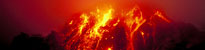# Jitter and sampling rateThe next step to advance our program is to include another measurement, the jitter. This is a useful measurement as it records how well our program and ADC are functioning.

Jitter is a measurement of the difference between the time taken for measurement and the true time for a device.

So our device can be set to take 16 samples per second, but in reality it may at first be 16.12, then 15.98, then 16.34. So it is slightly incorrect, and the differences (so 16.12 - 16 = 0.12; 0.12 is the jitter) are what is measured.

We will measure the sample rates so we can compare them to our set figure (look in the ADC set-up, there is a variable 'sps' - samples per second), which is 16.

## Programming

The start is very similiar to before. We must import the same libraries, set-up the ADC, although this time there is no need to make 'starttime' global, so only three things are declared.

import numpy
from obspy.core import Trace,Stream,UTCDateTime
import Queue
sps = 16 #samples per second
#this is how after how many samples a block is saved
block_length=128
#iterator for writing files
global block_id
block_id=0
#declare the q from library
queue = Queue.Queue()

The reading function has a few changes.

We don't need 'starttime' defined anymore. And inside the loop, we now use an array for 'sample' & 'sample_time', as we now need both, which will be queued instead. So we declare the array, fill it with the two values, and queue that.

for x in range (block_length):
#this array is for sample & sample_time
packet=[0,0]
timenow=UTCDateTime()
packet=sample
packet=timenow
print sample,timenow
queue.put(packet)

Next, the saving function, has some quite significant changes.

As before, this is a function, with an infinite loop inside, and inside that is the same if statement. Here it changes, now we declare two Numpy arrays, one for the samples, one for the jitter. Then we declare some variables, whose uses you will see next.

def save_data():
#iterator for writing files
global block_id
block_id=0
while True:
if queue.qsize() >= block_length:
#two numpy arrays for reading samples & jitter into
data=numpy.zeros([block_length],dtype=numpy.int16)
#note jitter uses float32 - decimals
jitter=numpy.zeros([block_length],dtype=numpy.float32)
firsttime=True
totaltime=0
sample_time = 0
sample_difference = 0

Next, while still in the if statement, as jitter is worked out by the differences from the last, the first sample cannot have a jitter value, as it has nothing to go from. Therefore the first sample must be got, and it gives no jitter value, only a 'previous_sample', to compare off. Just like the last time, it gets the queue value, but now it is an array.

#this is the loop without storing jitter value and calcs
packet = queue.get()
data = packet
starttime = packet
previous_sample=packet

Next, still in the if statement, we have the loop, which goes from 1 (not 0) to the block_length. It's 1 as we have sample 0 from just before. It gets the value from the queue, the sample is simply the value at location 0. We calculate the difference of the time of this value to the previous one. Then we have the jitter by taking the expected time from it, so we save it to the Numpy array. We store this value for next time, and total this up, for an average.

for x in range (1,block_length):
packet = queue.get()
data[x] = packet
sample_time=packet
sample_difference=sample_time- previous_sample #as sps is a rate, and s.d. is time, its 1 over sps jitter[x] = sample_difference - (1/sps)
#previous_sample is used to get the difference in the next loop
previous_sample=packet
totaltime=totaltime+sample_difference

We now have all the values in the NumPy arrays. So out of the loop, but still in the if statement, we calculate the average sample rate, for the MiniSeed header. So we can now fill in the header (with sampling rate now). Then, as before we stream and trace the data (there is no need to fill the header of the jitter, as it is not a seismograph. Then save them, note the jitter file is encoded as float32.

#a.s.r. is a rate, and t.t is time so its 1 over
avg_samplingrate = 1 / (totaltime/block_length)
stats = {'network': 'UK', 'station': 'RASPI', 'location': '00', 'channel': 'BHZ', 'npts': block_length, 'sampling_rate': avg_samplingrate,
'mseed': {'dataquality': 'D'},'starttime': starttime}
jitter_stream =Stream([Trace(data=jitter)])
#write block with id from iterator
sample_stream.write('mseed/MSEED' + str(block_id) + '.mseed',format='MSEED',encoding='INT16',reclen=512)
jitter_stream.write('mseed/JTR' + str(block_id) + '.mseed',format='MSEED',encoding='FLOAT32',reclen=512)
block_id += 1

Then identical to before, out of the loop and function (out of everything), we start the saving thread, and read data.

for x in range(5):

This should work now, and can be used to make seismographs. So you have a useful and working program.

## Next step... concatenating traces

We are not finished yet as the files it saves are not in an easy-to-find format, i.e. each block is saved separately. We need to combine the blocks into files containing a day's worth of blocks — see Concatenating traces.Earthquakes: how, why, where and when?
School seismology
FootballQuake
MarsQuake
News, teaching and museums